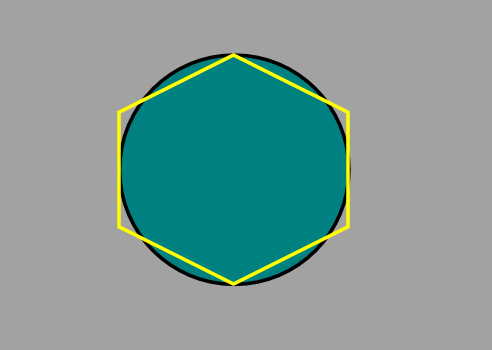# drawing a equal height and width hexagon

## Recommended Posts

I trying to create a "perfect" hexagon... that is in a fact a perfect hexagon for games, and I'm failing at it.

This is what I got so far

``````var game = new Phaser.Game(1024, 600, Phaser.AUTO, 'Phaser', {create: create, render: render});

var arr = [];
var graphics;
var cx = 300;
var cy = 200;
var size = 64;
var result;

function create() {

for(var c = 0; c < 6; c++){
arr[c] = hex_corner(cx, cy, size, c);
}

//draw vertices
for(var c = 0; c < 6; c++){
graphics.lineStyle(1, 0xffd900, 1);
if (c != 5){
graphics.moveTo(arr[c].x, arr[c].y);
graphics.lineTo(arr[c+1].x, arr[c+1].y);
}else{
graphics.moveTo(arr[c].x, arr[c].y);
graphics.lineTo(arr.x, arr.y);
}
}

//draw points
for(var c = 0; c < 6; c++){
graphics.beginFill(0xFF0000, 1);
graphics.drawCircle(arr[c].x, arr[c].y, 10);
}

result = 'height:' + (arr.y - arr.y) + ', width:' + (arr.x - arr.x)	;
}

function render(){
game.debug.text(result, 32, 32);
}

function hex_corner(center_x, center_y, size, i){
var coord = {};
var angle_deg = 60 * i   + 30 ;
var angle_rad = Math.PI/ 180 * angle_deg;

coord.x = center_x + size * Math.cos(angle_rad);
coord.y = center_y + size * Math.sin(angle_rad);

return coord;
}``````

The "edges" are not the same size, the width is not same and if I rotate it in the middle of a hex grid for instance it will be out of bounds.

So how do I make a perfect hexagon for any fixed size with equally sized edges, equal height and width?

This is what I would like to achieve. A hexagon with the corners outside of the circle.##### Share on other sites

``````function hex_corner(center_x, center_y, size, i){
var size2 = size + size/7;
var coord = {};
var angle_deg = 60 * i   + 30 ;
var angle_rad = Math.PI/ 180 * angle_deg;

if ( i==1 || i == 4) {
coord.x = center_x + size * Math.cos(angle_rad);
coord.y = center_y + size * Math.sin(angle_rad);
}else{
coord.x = center_x + size2 * Math.cos(angle_rad);
coord.y = center_y + size2 * Math.sin(angle_rad);
}
return coord;
}``````

This is what got me the closest to what I want, however it's still not as precise as I wish. It creates 4 point on the edges of a different sized circle. Any thoughts on how I can get the size of the 2nd circle to be more reliable?

##### Share on other sites

The solution if anyone is interested. This is in fact close enough for me, but if anyone else has other alternatives feel free to post it``````var game = new Phaser.Game(1024, 600, Phaser.AUTO, 'Phaser', {create: create, render: render});

var arr = [];
var graphics;
var cx = 300;
var cy = 200;
var size = 64;
var result;

function create() {

graphics.beginFill(0x0000FF, 1);
graphics.drawCircle(cx, cy, size*2);

//store point coordinates
for(var c = 0; c < 6; c++){
arr[c] = hex_corner(cx, cy, size, c);
}

//draw vertices
for(var c = 0; c < 6; c++){
graphics.lineStyle(1, 0xffd900, 1);
if (c != 5){
graphics.moveTo(arr[c].x, arr[c].y);
graphics.lineTo(arr[c+1].x, arr[c+1].y);
}else{
graphics.moveTo(arr[c].x, arr[c].y);
graphics.lineTo(arr.x, arr.y);
}
}

//draw points
for(var c = 0; c < 6; c++){
graphics.beginFill(0xFF0000, 1);
graphics.drawCircle(arr[c].x, arr[c].y, 5);
}

result = 'height:' + (arr.y - arr.y) + ', width:' + (arr.x - arr.x)	;
}

function render(){
game.debug.text(result, 32, 32);
}

function hex_corner(center_x, center_y, size, i){
//center_x , center_y are the coords for the center of the hexagon
//size  - first circle radius - used for generating 2 points on the circle
//size2 - 2nd circle radius - used to generate the edge that touch the 1st circle
var size2 = size + size/6.464;
var coord = {};
var angle_deg = 60 * i   + 30 ;
var angle_rad = Math.PI/ 180 * angle_deg;

//Check if the points should be placed on the 1st or 2nd circle
if ( i==1 || i == 4) {
coord.x = center_x + size * Math.cos(angle_rad);
coord.y = center_y + size * Math.sin(angle_rad);
}else{
coord.x = center_x + size2 * Math.cos(angle_rad);
coord.y = center_y + size2 * Math.sin(angle_rad);
}
return coord;
}``````

## Join the conversation

You can post now and register later. If you have an account, sign in now to post with your account.
Note: Your post will require moderator approval before it will be visible.×   Pasted as rich text.   Paste as plain text instead

Only 75 emoji are allowed.

×   Your previous content has been restored.   Clear editor

×   You cannot paste images directly. Upload or insert images from URL.